Science Journal of Applied Mathematics and Statistics
Volume 4, Issue 2, April 2016, Pages: 74-80

Implicit Exponentially Fitted RKNd Methods for Solving Oscillatory ODEs

Wenjuan Zhai1, *, Bingzhen Chen2

1Department of Mathematics, Beijing Jiaotong University Haibin College, Cangzhou, P. R. China

2Department of Applied Mathematics, Beijing Jiaotong University, Beijing, P. R. China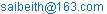(Wenjuan Zhai)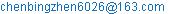(Bingzhen Chen)

*Corresponding author

Wenjuan Zhai, Bingzhen Chen. Implicit Exponentially Fitted RKNd Methods for Solving Oscillatory ODEs. Science Journal of Applied Mathematics and Statistics. Vol. 4, No. 2, 2016, pp. 74-80. doi: 10.11648/j.sjams.20160402.19

Received: February 25, 2016; Accepted: March 9, 2016; Published: April 13, 2016

Abstract: In this paper, we derive the implicit exponentially fitted RKNd methods for solving oscillatory ODEs. The new methods integrate exactly differential systems whose solutions can be expressed as linear combinations of functions from the set {exp(λt), exp(λt)}, λ C, or equivalently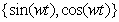when λ = iω, ω R. Numerical experiments are accompanied to show the efficiency and competence of the implicit exponentially fitted RKNd methods compared with implicit RKNd methods.

Keywords: RKNd Method, Exponentially Fitted, Implicit, Stability, Efficiency, Oscillatory

Contents

1. Introduction

A large number of problems arising in physical fields such as elastics, celestial mechanics, quantum differential equations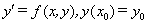(1)

In the begining, we use Euler method to solve these problems. But since Runge (1895) and Kutta (1905) derived the Runge-Kutta(RK) methods, we have constructed many RK methods of different orders (see Ref. [6,7]) to solve these problems. Bing-Zhen Chen and Xiong You gave a new class of method based on the analysis of the internal stage’s order of the RK method in . The new method is denoted as RKNd and when it comes to the initial value problem (1), it is a scheme of the form(2)

where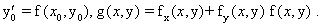Compared with the RK method, the RKNd method has two merits: (i) when they have the same stage number, RKNd method is able to achieve higher algebraic order than RK method; (ii) the internal stages ki of RKNd method have the same coefficients of h2 with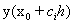. In addition, this scheme requires the function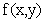of (1) has derivative of x. This restraint can’t be a problem in practice, because in most of the application problems the function has derivative or even higher derivative.

The RKNd method (2) can also solve the second order differential equations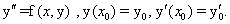(3)

If a new variable z is introduced to represent the first derivative y, then it is turned into the partitioned system of first order equations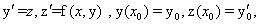So, we can use the RKNd method (2) to solve second order differential equations.

In the last decade, a great interest in the research of new methods for the numerical integration of initial value problems having oscillatory or periodic solutions has arisen. A problem is called oscillatory if the solution of the problem is oscillatory. When it comes to the second order initial value problems of the form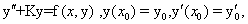(4)

where K is a symmetric positive semi-definite matrix (stiffness matrix) that contains implicitly the main frequencies of the problem, if the magnitude of the matrix K satisfies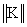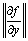, then the solutions of the initial value problem (4) are in general oscillatory or highly oscillatory. These initial value problems are of great interest in many problems of molecular dynamics, orbital mechanics, electronics, and so on, and they need efficient numerical methods because of the high accuracy demands.

Since Gautschi (see Ref. ) and Lyche (see Ref. ) gave the theoretical analysis of exponential fitting technic, many researchers have been working on the exponential fitting methods. A method is called exponentially fitted, that is to say, it integrates exactly differential systems whose solutions can be expressed as linear combinations of functions from the set{exp(λt), exp(λt), λ C}, or equivalently {sin(ωt), cos(ωt)} when λ=, ωR. The construction of exponentially fitted RK(N) methods is originally due to B. Paternoster (see Ref. ), and a detailed exposition of exponentially fitted methods with an extensive bibliography on this subject can be found in Ixaru and Vanden Berghe . And a lot of exponential fitting RK and RKN methods can be found in [3, 5, 11-14, 16]. When we solve oscillatory problems, if we can give a good estimate of oscillatory frequency, the exponential fitting method has an advantage over other methods. Therefore, it has become an indispensable tool for solving oscillatory problems.

Recently, Zhai Wenjuan and Chen Bingzhen has considered explicit EFRKNd methods in . In this paper, we construct the implicit exponentially fitted RKNd (IEFRKNd) methods based on the implicit RKNd methods derived in . The paper is organized as follows: In Section 1, we present the modified form of the RKNd method. Section 2 is concerned with the exponential fitting conditions of the modified RKNd method presented in Section 1. Section 3 is devoted to the algebraic order conditions of the modified EFRKNd method. In Section 4, we derive three two-stage implicit exponentially fitted RKNd methods of orders two, three and four respectively. In Section 5, we analyze the stability properties of the new methods, obtaining the stability regions. In Section 6, we present some numerical experiments to show the efficiency of the new methods compared with implicit RKNd methods given in .

2. Implicit Modified RKNd Methods

In this paper, we present a class of implicit modified RKNd methods which integrate exactly differential systems whose solutions can be expressed as linear combinations of the set of functions {exp(λt), exp(λt)} or equivalently {sin(ωt), cos(ωt)} when λ = , ω R. This means that the internal stage equations and the final step equation have to integrate exactly these sets of functions. So, we introduce a modification of the algorithm of implicit RKNd method (s 2), given by(5)

which can be expressed in the Butcher tableau form as.

We note that the algorithm (5) coincides with the algorithm of RKNd method (2) when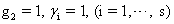, and the remaining coefficients are constant. So, the coefficients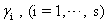and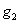are introduced in the algorithm definition so that the family of functions exp{±iωt}, can be integrated exactly by the method.

3. Exponentially Fitted Conditions

Following Albrecht’s approach (see Refs. [1,2]), the each stage of algorithm can associate a linear functional as follows.

for the internal stage(6)

for the final stage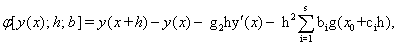(7)

Requiring that (6) and (7) will vanish for the functions from the set exp{±iωt}, we obtain the following equations:(8)

Having in mind the relations cosh(z) = (ez + ez)/2 and sinh(z) = (ez ez)/2, Eqs. (8) can be expressed in the form(9)(10)

The conditions defined by Eqs. (9) and (10) characterize when an implicit RKNd method (5) is exponentially fitted, and therefore they will be denominated as exponential fitting conditions (EF conditions).

4. Algebraic Order Conditions

Now, we make a study of the local truncation error for the IEFRKNd methods in order to obtain the order conditions for the algorithm (5).

As discussed in , we consider the following assumptions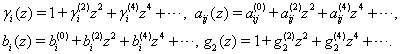(11)

Using the assumptions mentioned above and following the way given in Hairer [6, pp.143-148] for obtaining the terms of the local truncation error, the order conditions for IEFRKNd methods (up to fifth order) are the following ones:

Order 2 requires: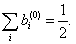(12)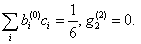(13)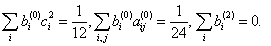(14)(15)

5. Construction of Implicit EFRKNd Methods

In this section, we analyze the construction of implicit two-stage EFRKNd methods (up to order 4) with the help of the order conditions obtained in the previous section.

In order to derive IEFRKNd methods corresponding to IRKNd methods obtained in , we assume the coefficients satisfy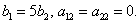So the EF conditions (9) and (10) reduce to(16)(17)

From (16) and (17), we have(18)

The taylor expansions of these coefficients areWe can verify that the coefficients are satisfied the second order condition (12). So the IEFRKNd method has algebraic order at least two.

As we can see, when z → 0, the method (18) reduces to IRKNd method in . We can choose different values of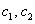to obtain different order methods. As discussed in , we can obtain the following different order methods.

First, we choose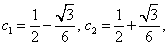then (18) becomesWe denote this method as IEFRKNd2s2. When z → 0, it reduces to the method IRKNd2s2. We can verify that the method IEFRKNd2s2 is of order 2.

Second, by choosing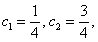(18) becomesWe denote this method as IEFRKNd2s3. When z → 0, it reduces to the method IRKNd2s3. We can verify that the method IEFRKNd2s3 is of order 3.

Now we derive the fourth order method. From the order conditions (13) and (14), we obtain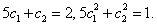So,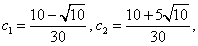and we obtain the fourth order method also obtain a fourth order IRKNd methodWe denote this method as IEFRKNd2s4. Here, we also obtain a fourth order IRKNd method.

6. Stability Properties and Phase Analysis

Before we put a method into application, we must analysis its stability or it will be dangerous. Roughly speaking, the stability of a numerical method is that the numerical solution has a small change when the initial value has a small perturbation. Since the coefficients of exponentially fitted method depend on z = λh, we consider its imaginary stability region (see Ref. ).

Applying the method (5) to the test equation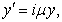we obtain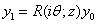, whereθ = µh, i2= 1, and R(; z) is called the stability function. The region of imaginary stability is a region in the θ z plane (θ > 0, z > 0), throughout which | R(; z) |≤ 1. In Fig. 1, (a)- (e) show the stability regions for IEFRKNd2s2, IRKNd2s2, IEFRKNd2s3, IRKNd2s3 and IEFRKNd2s4 respectively.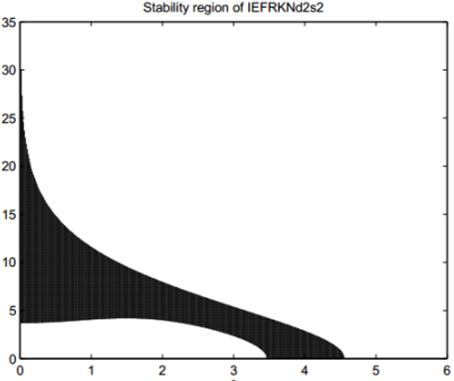(a)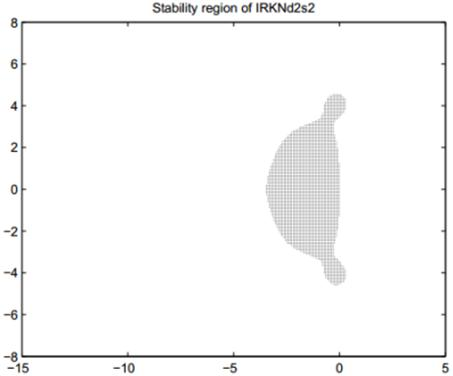(b)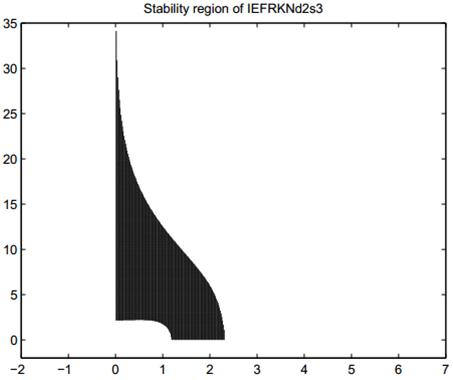(c)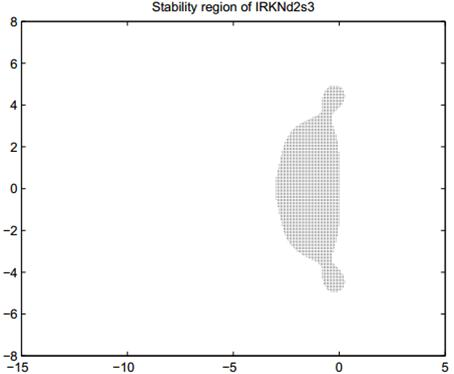(d)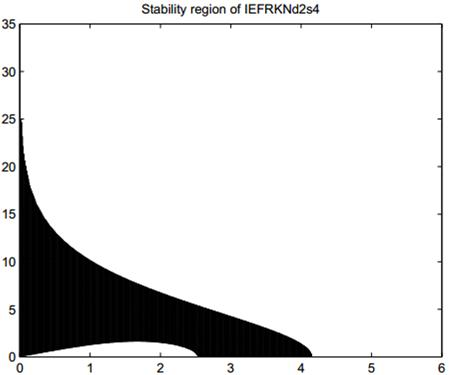(e)

Fig. 1. Stability regions for the methods IEFRKNd2s2 (a), IRKNd2s2(b), IEFRKNd2s3 (c), IRKNd2s3(d), IEFRKNd2s4 (e).

From Fig. 1, we can see our IEFRKNd methods have larger stability regions than IRKNd methods.

7. Numerical Experiments

To test the numerical performance of the implicit EFRKNd methods, we carry out experiments on three oscillatory or periodic problems to illustrate the effectiveness and efficiency. The codes used in the comparisons are:

IEFRKNd2s2: two-stage IEFRKNd method of order 2 derived in this paper;

IEFRKNd2s3: two-stage IEFRKNd method of order 3 derived in this paper;

IEFRKNd2s4: two-stage IEFRKNd method of order 4 derived in this paper;

IRKNd2s2: two-stage IRKNd method of order 2 given in ;

IRKNd2s3: two-stage IRKNd method of order 3 given in ;

The criterion used in the numerical comparisons is the decimal logarithm of the maximum global error (log10(GE)) versus the computational effort measured in the number of function evaluations required by each method.

Problem 1. Consider the second order ODE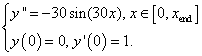whose analytic solution is given by y(x) = sin(30x)/30.

In our test we chose the parameter values ω = 30, λ = 30i, xend = 100, and the numerical results presented in Figure. 2(a) have been computed with the integration steps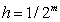, m =1, 2, 3, 4, 5.

Problem 2. Consider the inhomogeneous equation (see Ref. )

y′′ + 100y = 99 sin(x), y(0) = 1, y= 11(11),

whose analytic solution is given by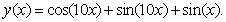The equation has been solved in the interval [0, 10] with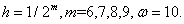The numerical result is shown in Fig. 2 (b).

Problem 3. Consider the nonhomogeneous Duffing equation used in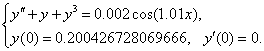The ‘exact’ solution was first given by Van Dooren (see Ref. ), but a more accurate solution was given by Zhao et al. (see Ref. ), i.e.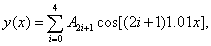withThe equation is integrated over the interval [0, 100] with, m = 1, 2, 3, 4, ω = 1.01. The numerical result is shown in Fig. 2(c).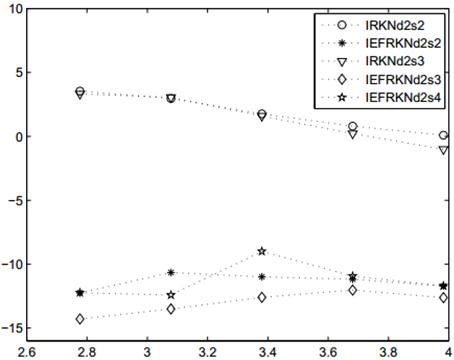(a)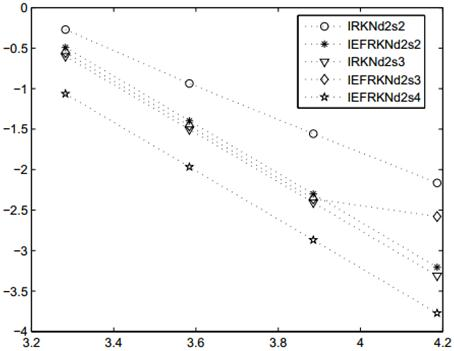(b)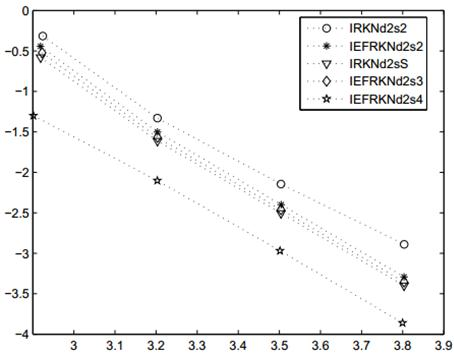(c)

Fig. 2. Efficient curves for Problem 1 (a), Problem 2 (b), Problem 3 (c).

From Fig. 2(a), we can see that when the solution of differential system can be expressed as linear combinations of functions from the set {exp(λt), exp(λt)}, λ C, our EF IRKNd methods are more efficient than IRKNd methods which are not exponentially fitted. From Fig. 2(b) and Fig. 2(c), we can find that the efficient curves of the methods IEFRKNd2s2, IEFRKNd2s3 and IRKNd2s23 are almost same. But the method IEFRKNd2s4 is the most efficient method among them.

8. Conclusion

In this paper, we derive the implicit exponentially fitted RKNd methods of different order for solving oscillatory ODEs. These new methods integrate exactly differential systems whose solutions can be expressed as linear combinations of functions from the set {exp(λt),exp(λt)}, λ C, or equivalentlywhen λ = iω, ω R. We also analysis the stability of these methods. Numerical experiments are accompanied to show the efficiency and competence of the implicit exponentially fitted RKNd methods compared with implicit RKNd methods.

References

1. P. Albrecht, The extension of the theory of A-methods to RK methods. In: Strehmel, K. (Ed.), Numerical Treatment of Differential Equations, Proceedings of the 4th Seminar NUMDIFF-4, Tuebner-Texte Zur Mathematik. Tuebner, Leipzig 1987, 8-18.
2. P. Albrecht, A new theoretical approach to Runge Kutta methods,SIAM J. Numerical Anal., 1987, 24: 391-406.
3. Blanes S. Explicit symplectic RKN methods for perturbed non-autonomous oscillators: Splitting, extended and exponentially fitting methods,Computer Physics Communications, 2015, 193:10-18.
4. B. Zh. Chen and X. You. RKNd methods for solving initial value problems,Numer. Math. Sinica., 2010, 32(4): 399-412. (in Chinese).
5. D’Ambrosio R, Paternoster B, Santomauro G. Revised exponentially fitted Runge–Kutta–Nyström methods,Applied Mathematics Letters, 2014, 30(30):56-60.
6. E. Hairer, S P. Nφrsett and G. Wanner, Solving ordinary differential equations I, Nonstiff problems [M]. Berlin: Springer-Verlag 1993.
7. J C. Butcher, The Numerical Analysis of Ordinary Differential Equations [M]. Wiley: Chichester UK 1987.
8. W. Gautschi, Numerical integration of ordinary differential equations based on trigonometric polynomials,Numer. Math., 1961, 3: 381-397.
9. T. Lyche, Chebyshevian multistep methods for ordinary differential equations,Numer. Math., 1972, 19: 65-75.
10. B. Paternoster, Runge-Kutta(-Nyström) methods for ODEs with periodic solutions based on trigonometric polynomials,Appl. Numer. Math., 1998, 28: 401-412.
11. G. Vanden Berghe, H. De Meyer, M. Van Daele and T. Van Hecke, Exponentially fitted Runge-Kutta methods,J. Comp. Appl. Math., 2000, 125: 107-115.
12. J. M. Franco, An embedded pair of exponentially fitted explicit Runge-Kutta methods,J. Comput. Appl.Math., 2002, 149: 407-414.
13. J. M. Franco, Exponentially fitted explicit Runge-Kutta -Nyström methods,J. Comput. Appl. Math., 2004, 167: 1-19.
14. J.M.Franco,I. Gómez,Symplectic explicit methods of Runge–Kutta–Nyström type for solving perturbed oscillators. Journal of Computational & Applied Mathematics, 2014, 260:482-493.
15. L. Gr. Ixaru and G. Vanden Berghe, Exponential Fitting, Kluwer Academic Publishers, Dordrecht, 2004.
16. T.Monovasilis,Z.Kalogiratou, T E.Simos,Construction of Exponentially Fitted Symplectic Runge–Kutta–Nyström Methods from Partitioned Runge–Kutta Methods. Mediterranean Journal of Mathematics, 2014, 1618(1):1-15.
17. T. E. Simos and J. Vigo-Aguiar, Exponentially fitted symplectic integrator,Phys. Rev. E., 2003, 67: 1-7.
18. H. Van de Vyver, Runge-Kutta type methods for periodical initial value problems, PhD thesis, Catholic University of Leuven, 2006.
19. Hans Van de Vyver, A Runge-Kutta-Nyström pair for the numerical integration of perturbed oscillators,Comput. Phys. Commun., 2005, 167: 129-142.
20. R. Van Dooren, Stabilization of Cowell’s classical finite difference method for numerical integration,J. Comput. Phys., 1974, 16: 186-192.
21. W. J. Zhai, B. Zh. Chen, Exponentially Fitted RKNd Methods for Solving Oscillatory ODEs, Advances in Mathematics, 2013, 42(3): 393-403.
22. D. Zhao, Z. Wang and Y. Dai, Importance of the first-order derivative formula in the Obrechkoff methods,Comput. Phys. Commun., 2005, 167: 65-75.

 Contents 1. 2. 3. 4. 5. 6. 7. 8.
Article ToolsAbstractPDF(795K)